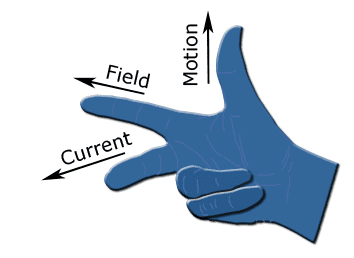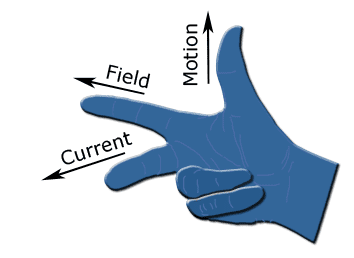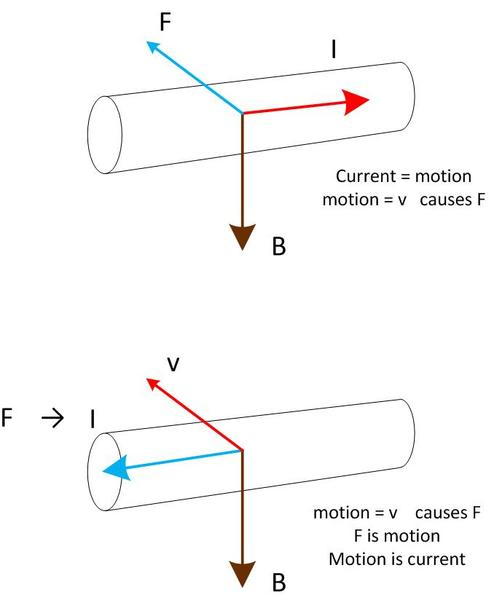# Fleming's left and Right hand rule

Gold Member

## Homework Statement

Not a Home-work problem,just a doubt.Since this is a school doubt,I will post here.

Fleming's left and right hand rule
Why do we use two hands for the same thing?Can't we just use one hand?
Why is it different?

For example,Magnetic field direction is to right.Current is moving into the Monitor(Cross)
Applying the left hand rule,wire will move up.
Applying right hand rule,wire will move down.
What's the difference?They are both the same thing,isn't it?

BruceW
Homework Helper
your example is not quite correct. Using the left hand rule, you must have your index finger representing the magnetic field, your middle finger represents current, and your thumb represents the force on the wire. So, by the left-hand rule for your example, the wire will move down.
And for the right-hand rule, you must use the current as your index finger, the magnetic field as your middle finger, and the force as your thumb. So again, the wire will move down. So yes, both rules are essentially saying the same thing. This webpage gives a nice explanation: http://en.wikipedia.org/wiki/FBI_mnemonics

Also, you can use any cyclic permutation, and it will still give you the right answer for the directions. So if we say (a,b,c) means (thumb, index finger, middle finger) then using the right-hand rule, we can use (F,I,B) OR (I,B,F) OR (B,F,I) (where F is force, I is current, and B is magnetic field). Note that (F,I,B) is the most commonly used way to use the right-hand rule. But you can use any of these ways, and you get the correct directions.

So, also, if we again say (a,b,c) means (thumb, index finger, middle finger), then using the left-hand rule, we can use (F,B,I) OR (B,I,F) OR (I,F,B) Any of these methods will give you the correct directions, But (F,B,I) is the most commonly used one (which is called the 'FBI rule' if you read the wikipedia page). If you are familiar with cross-product, it will make more sense. But if you haven't learned about cross product yet, then that is something to look forward to :)

Now, having said all that, sometimes people use the right-hand rule to talk about the velocity of a wire moving through a magnetic field, and the induced current. This case is different from the previous case, because in the previous case, we were considering a wire which already has current going through it, and the force on that wire due to the magnetic field.

When people talk about the induced current due to a wire moving through a magnetic field, we must use the rule (v,B,I) for the right-hand (i.e. velocity of the wire is the thumb, magnetic field is the index finger and induced current is middle finger). Now we can also use a left-hand rule for this case if we want to. For the left-hand, we must use the rule (B,v,I) so using left-hand, the magnetic field is the thumb, the velocity of the wire is the index finger, and the induced current is the middle finger. And also, a cyclic permutation will also work.

BvU
Homework Helper
Hello good friend,

If you find this handedness confusing, you can always fall back to the corkscrew rule for cross products: ## \hat x \wedge \hat y = \hat z## : turn ##\hat x## over the smallest angle towards ##\hat y##. ## \hat z## points the way a corkscrew goes.

Try to remember all this vector- and pseudo-vector related stuff as cross products.
Math from there on.
permutations: you can cycle -- that's why FIB = IBF --,
you can swap if you insert a minus sign,
cartesian coordinates: the determinant way of writing the cross product,
etc. etc.
Not only for vectors like ## \vec F, \vec B## but also for ##\vec \nabla##.​

And try to avoid lefthand formulations, they only make life more difficult. Lorentz force is ##\vec F = q\ \vec v \wedge \vec B## should be etched in memory. From there the direction of a force on a current is obvious.

Personally I find the wiki link completely objectionable. Left hand as mentioned above, right hand FIB confusing. IBF is the only accceptable one. The wrap-around (rh grip rule) for ##\vec B## so-so. I much prefer ## d\vec B = k {d\vec I \wedge \vec r \over |\vec r|^2}##

•1 person
Yes you can use just one hand for both. It is much better to use the right hand to give the direction of a magnetic field around a conductor which you can then visualise interacting with the field from the magnets. It is much better to just use the right hand for the vector cross product relationship F = q v cross B from which you can derive F = l i cross B. Don't use F=Bil it points in the wrong direction.

There is no need for the left hand rule. No need to make a distinction between generators and motors: both are electromagnetic machines, both obey the same laws of physics.

[edit: Ninja'd]

Gold Member
And for the right-hand rule, you must use the current as your index finger, the magnetic field as your middle finger, and the force as your thumb.
Really?
Then what is this?Thank you all.
btw,I haven't learned vector products yetBvU said:
Hello good friendGold Member
Anyone?

BvU
Homework Helper
I haven't learned vector products yet
Well, you have now: See post #3 for the direction, ##\vec z \bot## both ##\vec x ## and ##\vec y##. Magnitude: |z| = |x| * |y| * ##\sin\theta## where theta is the angle from x to y.

Picture in your last post is a right hand. And I see no contradiction with Bruce; what is your reason for the question mark ?

Gold Member
Well, you have now: See post #3 for the direction, ##\vec z \bot## both ##\vec x ## and ##\vec y##. Magnitude: |z| = |x| * |y| * ##\sin\theta## where theta is the angle from x to y.
I still don't understand.What is z,x and y?
Picture in your last post is a right hand. And I see no contradiction with Bruce; what is your reason for the question mark ?
What BruceW said:
BruceW said:
And for the right-hand rule, you must use the current as your index finger, the magnetic field as your middle finger, and the force as your thumb.
What the picture says:
Picture said:
Index finger is the Field.Middle finger is the current
This is the question markBvU
Homework HelperIs something to do with motors NO generators!. These dumbo mechanical and electrical engineers need one rule for motors and another for generators. It's really stupid. Now they need another mnemonic to remember which is for which. Defeats the purpose altogether.
Stick to right hand. Remember the vector equations. Begin etching the Lorentz eqn in your memory.

And: if you don't know about cross products, or outer products, you can stay on the same google page.

Gold Member
Remember the vector equations. Begin etching the Lorentz eqn in your memory.
Let's forget about Lorentz transformations(Or whatever)
Just tell me why that picture is wrong.
My text book has the same picture too.Index is the field.Middle is the current.
Right hand is used to find the direction of current when a conductor moves in a magnetic field(Generator)
Left hand is used to find the direction of force when a current moves in a magnetic field.(Motor)BvU
Homework Helper
It's not wrong in the sense that it is incorrect or something. But if you have to remember two things (left hand, right hand) in order not to mix up two other things (motor, generator), then all you do is shift the difficulty to not mixing up the two things (which hand was it again for motors ? for generators?).

If your textbook does it this way, you're probably better off just accepting it. I'm glad I didn't have to go ambidextrous ..

The Lorentz force is for charges in electromagnetic fields. "magnetic field" was in your OP (#). If you don't know about cross products, you certainly don't have to know about Lorentz transformations yet.

(#) coming back to that: maybe this two hand jumble uses this roundabout way to distinguish between one case where B and I cause F and in the other where F and B cause I. Really don't know.

BruceW
Homework Helper
Really?
Then what is this? ...
the picture says index finger is field, middle finger is induced current, velocity (of the wire) is thumb. This is exactly as I said near the end of my post:
BruceW said:
When people talk about the induced current due to a wire moving through a magnetic field, we must use the rule (v,B,I) for the right-hand (i.e. velocity of the wire is the thumb, magnetic field is the index finger and induced current is middle finger). Now we can also use a left-hand rule for this case if we want to. For the left-hand, we must use the rule (B,v,I) so using left-hand, the magnetic field is the thumb, the velocity of the wire is the index finger, and the induced current is the middle finger. And also, a cyclic permutation will also work.
You need to distinguish between two cases 1) force on a wire due to it having current and being affected by a magnetic field. 2) induced current in a wire due to velocity of the wire through a magnetic field. In reality, both of these cases can be explained by one law (the Lorentz force law). But if you're not familiar with that, then you need to remember the two cases, and what rule to use for each.

Gold Member
I still don't understand.Let's start from the first post.
Magnetic field is to the [STRIKE]left[/STRIKE] right.Current is going into the monitor.
According to the left hand rule,Wire will move down.

Now we will stop the current and move it down manually.Now according to right hand rule,induced current moves out from monitor.
?????

We did the same thing .Why is it showing different answers?

btw,you are fast BvU! :surprised

Last edited:
BruceW
Homework Helper
I think you meant to say Magnetic field is to the right. If you did mean this, then yes, both of your statements are correct.

But we did not do the same thing. This is a crucial point. In one case, we get a force on the wire due to a current going into the monitor. The other case is that we are manually moving the wire down, and we get induced current going out of the monitor. An EM force on wire due to a current is not the same thing as an induced current due to movement of a wire through a magnetic field. In a certain sense, they are opposite things. And that is why the current is in opposite directions for the two cases.

Gold Member
I think you meant to say Magnetic field is to the right. If you did mean this, then yes, both of your statements are correct.
Oh sorry.

An EM force on wire due to a current is not the same thing as an induced current due to movement of a wire through a magnetic field. In a certain sense, they are opposite things. And that is why the current is in opposite directions for the two cases.
Can you tell me why is that?

BvU
Homework Helper
Good reaction: do not accept explanations just out of politeness if you don't really understand them. I mean it. You just might make friends yet! And it enticed me to sort it out (for my own sake just as well, so no need to thank me; I should be thanking you...) in an understandable way.

To keep a long story short I add over a Thousand words:Big picture, big importance (imho)

Both pictures:
• Positive ##x## direction is to the right
• Magnetic field is down. Consider that the positive ##y## direction• Big arrowheads: in the ##x\, y## plane
• Smaller arrowheads in the ##\hat z = \hat x \times \hat y## direction (a very simple cross product)
• The coordinate system is right-handed
• The Lorentz force is ##\vec F = q\, \left (\vec E + \vec v \times \vec B \right )##
• There is no E field
• Right hand rule: in ##\vec a \times \vec b = \vec c##
a thumb
b index
c middle -- points in the direction of c​
• Corkscrew rule: in ##\vec a \times \vec b = \vec c##
turn ##\vec a## over the smallest angle towards ##\vec b## : Corkscrew goes in the direction of c​

Top picture:
• We see a wire carrying current to the right. Current is moving charge. Moving charge has a "velocity".
• We consider mobile positive charges in the wire• The Lorentz force is ##\vec F = q\, \vec v \times \vec B ##
• All moving positive charges in the wire feel this Lorentz force
• Together they "push" in the direction of ##\vec v \times \vec B ##
• mutatis mutandis for moving negative charges: they move in the negative x-direction and the resulting Lorentz force points in exactly the same direction (- q x -v = +q x +v)

Bottom picture:
• We see a wire being dragged in the positive ##z## direction with a velocity ##\vec v##.
• We consider mobile positive charges in the wire• The Lorentz force is ##\vec F = q\, \vec v \times \vec B ##
• All moving positive charges in the wire feel this Lorentz force
• Together they "push" in the direction of ##\vec v \times \vec B ##, which is the negative ##x## direction
• This "push" is an electromagnetic potential (emf) that can cause a current.

That's all, folks!

A few hints and tips for the diehards:
My Post #3 is well-meant advice. I think NoPoke backs me up there. It serves me well even now.
I notice I use the \wedge and the \times for the cross product. Sorry. For Math the wedge has another meaning (as well?). Context generally helps lift ambiguity.
Getting by ignoring the left hand everything avoids all confusion altogether. Very effective.

•1 person
BruceW
Homework Helper
yeah, I agree with BvU's pictures. Also, I think it helps to think in terms of each of the individual free charge carriers inside the wire. And this is explained by the Lorentz force law. I guess you don't really need to know about cross-product to understand the idea qualitatively. In the Lorentz force law, (using right hand) the motion of charge carriers is the index finger, the middle finger is the magnetic field, and the thumb is the force on the charge carrier.

p.s. note that I've used the phrase 'charge carriers', but specifically, I've been talking about positive charge carriers. for negative charge carriers, to use the Lorentz force law, you just reverse the direction of motion of the charge carriers. But in either case, if you are just given 'current', this essentially means "the direction of motion if the charge carriers were positive" So if you are given the current, you don't need to worry about whether the charge carriers are positive or negative. And similarly, if you are trying to calculate the induced current, due to motion of the wire, this does not depend on the sign of charge of the charge carriers.

So, in the case where there is no current in the wire, and we move the wire down manually, the charge carriers in the wire are moving downwards, and if the magnetic field is moving to the right, there will be a force on the charge carrier, which pushes it out of the screen, towards us. So there is an induced current coming out of the screen. And in the case when there is current going into the screen, and the wire has no movement, this means each of the charge carriers is moving into the screen, and the magnetic field is to the right, so the force on the charge carriers is down. But, the charge carriers can't move down, because they are inside the wire, so they force the entire wire to move down with them.

•1 person
Gold Member
Thank you guys.Thank you for the loong post BvUI guess I will need to learn vectors more deeply(And of course Lorentz transformation(Force or whatever)).I have just started vectors(Last Sunday).Anyway,when will I learn this Lorentz force? I'm in grade 10.

The basic reason that we have two rules, i think, is because we conventially take a current direction as opposite to the actual current carriers i.e electrons.

Last edited: